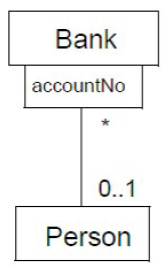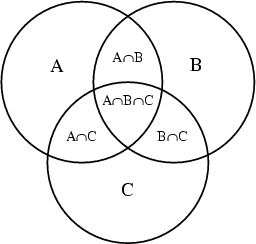# Logical Data Modeling - Association

An association is a relationship between two or more entities. It's represented by a link in the data model.

An association:

• answers to the question why two entity need to know about one another.
• specifies a semantic relationship (ie is … of) that can occur between entities.

## Example

• A mathematical function y = f(x) creates an association relationship between the set of x and the set of y
• A book has been written by one or many authors.

## Properties

The following relationship properties are visible:

And an association may also have a type information added with a symbol at one of its end such as:

As the term association design a visual representation of an relationship, the term association is also sometimes combined with the relationship properties.

Example:

## Visualization

### Entity Diagram

Example of Entity Diagram

where:

• PublishedBy is an association where
• 1 is the multiplicity of the publisher end and indicates that a book is published by one publisher
• * is the multiplicity of the book end and indicates that a publisher publishes many books
• WrittenBy is an association
• * is the multiplicity of the author end and indicates that a book is written by many Author
• * is the multiplicity of the book end and indicates that a author writes many books

### Class Diagram

• Any Association is drawn as a diamond with a solid line to the entity (class).
• The Association’s name can be shown as a name string near the Association symbol
• An Association end is the connection between the line depicting an Association and the icon (often a box) depicting the class.
• The multiplicity attached to a class end denotes the possible cardinalities of the set of entity.

The figure shows:

• a binary association: where the solid triangle indicates the order of reading: Player PlayedInYear Year
• a ternary association: between Team, Year, and Player with id (ends) named team, season, and goalkeeper (goalie) respectively.

Recommended Pages
Logical Data Modeling - Aggregation (Collection)

aggregation is a containment relationship where one or more entities are part of a container entity. In other word, aggregation is combining multiple pieces of data into one unit. The container entity...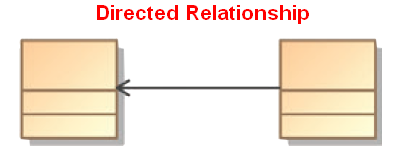Logical Data Modeling - Antisymmetry relationship

A Antisymmetric relation is a relationship that happens when for all a and b in X: if a is related to b then b isNOT related to a or b=a (reflexivity is allowed) In mathematical notation, an Antisymmetric...Logical Data Modeling - Asymmetric Relation (Uni-directional|Antisymmetric)

An asymmetric relation is a type of binary relation that requiers: antisymmetry (ie if a is related to b, b is not related to a) and irreflexivity (ie an element cannot be related to itself) irreflexivity...Logical Data Modeling - Classification (Taxonomy | Categorization) - Transversal Data Organization

Classification is an naming technique for organization where entity or relationship gets classified by giving them a nominal attribute known as a classifier. relationshipsgroups or categories The output...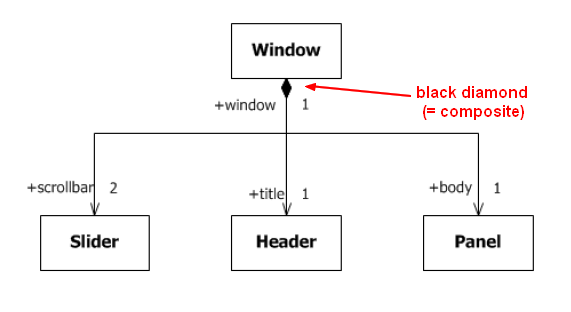Logical Data Modeling - Composition Relationship

composition is a containment relationship of physical type where the content entity is a part of a container entity This relationship is also known as: a whole/part relationship (ie whole/component)...Logical Data Modeling - Relationship

A relationship is a model element that relates two entities . In its simple form (semantic form), it's a description that has a verb such as: has consists of uses ... entityentity setrelationship...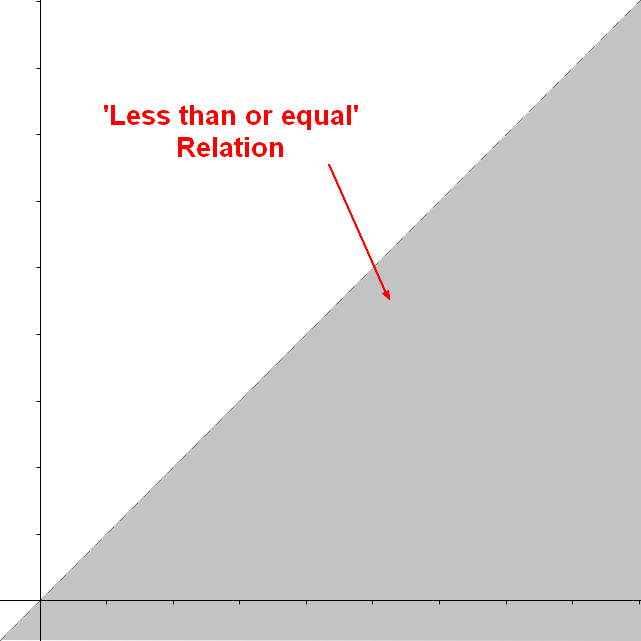Logical Data Modeling - Relationship Representation / Implementation / Visualisation

A relationship can be described/implemented by: a function a a a a A relationship can be represented as a function Example with a three element (ternary) relationship: A binary...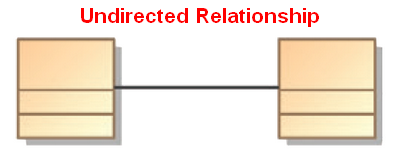Logical Data Modeling - Symmetric relationship (bi-directional)

A symmetric relation is a type of binary relation that happens when for all a and b in X if a is related to b, then b is related to a In mathematical notation, the relation f between x and y is...